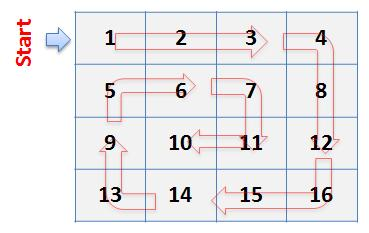### C++ Program to print a matrix in Spiral order

Spiral order is an order especially used in 2-D Matrices or 2 dimensional matrices in which we have both rows and columns. In spiral order we print the matrix in a spiral form such as shown in the below figure.As shown in the above figure we start printing the matrix from 1st row then move to the last column and then print the last row and then to the first column such as drawing a boundary to the inner sub-matrix then we move on to the inner sub-matrix until the whole matrix isn't printed.
Let us assume that we have given a matrix of size n*m as input that is having n rows and m columns .In order Algorithm :1.to print the spiral order of the matrix we use 4 variables or we require 4 variables.
1st variable which points the element where the  row order has been started . row_start=0 since elements in the array start from index:0.
2nd variable which points the element where the  row order has been ended. row_end=0 since elements in the array start from index:0 and ends at index:n-1.
3rd variable which points the element  from where the  column order has been started. column_start=0.
4th variable which points the element   where the  column order has been ended. column_end=0.
here we have 4 traversals for every boundary or for every spiral traversal that is from row_start to column_start , column_start to row_end ,row_end to column_end ,column_end to row_start.
2. First of all, we will traverse in the row row_start from column_start
to column_end and we will increase the row_start with 1 as we have
traversed the starting row.
3. Then we will traverse in the column column_end from row_start to
row_end and decrease the column_end by 1.
4. Then we will traverse in the row row_end from column_end to
column_start and decrease the row_end by 1.
5. Then we will traverse in the column column_start from row_end to
row_start and increase the column_start by 1.
6. We will do the above steps from 2 to 5 until row_start <= row_end
and column_start <= column_end.

Program:
#include <iostream>

using namespace std;

int main()
{
int n,m,i,j;
cout<<"enter the size of the 2-d array";
cin>>n>>m;
int a[n][m];
//reading the elements into the array
for(i=0;i<n;i++){
for(j=0;j<m;j++){
cin>>a[i][j];
}
}
//spiral order printing
int row_start=0,row_end=n-1,column_start=0,column_end=n-1;
while(row_start<=row_end&&column_start<=column_end){
//for row_start
for(int col=column_start;col<=column_end;col++){
cout<<a[row_start][col]<<" ";
}
row_start++;
//for column_end
for(int row=row_start;row<=row_end;row++)
cout<<a[row][column_end]<<" ";
column_end--;

//for row_end
for(int col=column_end;col>=column_start;col--)
cout<<a[row_end][col]<<" ";
row_end--;
//for column_start
for(int row=row_end;row>=row_start;row--){
cout<<a[row][column_start]<<" ";
}
column_start++;
}

return 0;
}
Sample input:
enter the size of the 2-d array 4 4
1 2 3 4
5 6 7 8
9 10 11 12
13 14 15 16
Sample output:
1 2 3 4 8 12 16 15 14 13 9 5 6 7 11 10#### More Articles of Shaik Aftab Ahmed:

Name Views Likes
C++ Program to Find the Frequency of Odd & Even Numbers in the given Matrix 394 1
C++ program to Sort a Linked List Using Merge Sort 358 1
C++ Program to Implement a Linked List representation of a Binary tree 353 1
C++ Program to Check for balanced parentheses in an expression 251 1
C++ Program to Perform Inorder, Preorder, Postorder traversals in a binary tree. 289 1
C++ program to print Indian flag 385 1
C++ program to Convert a multi level linked list to a singly linked list 269 1
C++ program to print right view of a Binary tree using queue 244 1
C++ Program to implement Huffman Coding Compression Algorithm 1660 1
C++ Program to Create a Height Balanced Binary Tree 274 1
C++ program to implement Prims algorithm 635 1
C++ Program for BFS Traversal 289 1
C++ Progam to Evaluate a Prefix Expression 460 1
C++ Program to Implement Queue using Linked List 254 1
C++ implementation of Stack using Linked list 303 1
C++ program to find the intersection point of two linked lists 340 1
C++ program to count the inversions in the given array 278 1
C++ program to perform D.N.F sort 325 1
C++ program to print all possible subsets of a String 286 1
C++ program to count the number of ones in a binary representation of a number 310 1
C++ program to print all possible subsets of a set 322 1
C++ program to find the largest word in a String 287 1
C++ Program to print a matrix in Spiral order 366 1
C++ program to convert from Binary to Decimal, Octal to Decimal, Hexadecimal to Decimal 313 1
C limits library 340 1
Program to add two Binary numbers 332 1